# Formulating Algorithms: Counter-Controlled Repetition

### Formulating Algorithms Counter Controlled Repetition

To illustrate how algorithms are developed, we modify the GradeBook class of Chapter 4 to solve two variations of a problem that averages student grades. Consider the following problem statement:

A class of 10 students took a quiz. The grades (integers in the range 0 to 100) for this quiz are available to you. Determine the class average on the quiz.

The class average is equal to the sum of the grades divided by the number of students. The algorithm for solving this problem on a computer must input each grade, keep track of the total of all grades input, perform the averaging calculation and print the result.

Pseudocode Algorithm with Counter-Controlled Repetition

Let's use pseudocode to list the actions to execute and specify the order in which they should execute. We use counter-controlled repetition to input the grades one at a time. This technique uses a variable called a counter (or control variable) to control the number of times a set of statements will execute. Counter-controlled repetition is often called definite repetition, because the number of repetitions is known by the application before the loop begins executing. In this example, repetition terminates when the counter exceeds 10. This section presents a fully developed pseudocode algorithm (Fig. 5.5) and a version of class GradeBook (Fig. 5.6) that implements the algorithm in a C# method. The section then presents an application (Fig. 5.7) that demonstrates the algorithm in action. In Section 5.9, we demonstrate how to use pseudocode to develop such an algorithm from scratch.

Figure 5.5. Pseudocode algorithm that uses counter-controlled repetition to solve the class-average problem.

 ``` 1 set total to zero 2 set grade counter to one 3 4 while grade counter is less than or equal to ten 5 prompt the user to enter the next grade 6 input the next grade 7 add the grade into the total 8 add one to the grade counter 9 10 set the class average to the total divided by ten 11 print the class average ```

Figure 5.6. GradeBook class that solves the class-average problem using counter-controlled repetition.

(This item is displayed on pages 185 - 186 in the print version)

 ``` 1 // Fig. 5.6: GradeBook.cs 2 // GradeBook class that solves class-average problem using 3 // counter-controlled repetition. 4 using System; 5 6 public class GradeBook 7 { 8 private string courseName; // name of course this GradeBook represents 9 10 // constructor initializes courseName 11 public GradeBook( string name ) 12 { 13 CourseName = name; // initializes courseName by using property 14 } // end constructor 15 16 // property to get and set the course name 17 public string CourseName 18 { 19 get 20 { 21 return courseName; 22 } // end get 23 set 24 { 25 courseName = value; // set should validate 26 } // end set 27 } // end property CourseName 28 29 // display a welcome message to the GradeBook user 30 public void DisplayMessage() 31 { 32 // property CourseName gets the name of the course 33 Console.WriteLine( "Welcome to the grade book for {0}! ", 34 CourseName ); 35 } // end method DisplayMessage 36 37 // determine class average based on 10 grades entered by user 38 public void DetermineClassAverage() 39 { 40 int total; // sum of the grades entered by user 41 int gradeCounter; // number of the grade to be entered next 42 int grade; // grade value entered by the user 43 int average; // average of the grades 44 ``` 45 // initialization phase 46 total = 0; // initialize the total 47 gradeCounter = 1; // initialize the loop counter 48 49 // processing phase 50 while ( gradeCounter <= 10 ) // loop 10 times 51 { 52 Console.Write( "Enter grade: " ); // prompt the user 53 grade = Convert.ToInt32( Console.ReadLine() ); // read grade 54 total = total + grade; // add the grade to total 55 gradeCounter = gradeCounter + 1; // increment the counter by 1 56 } // end while 57 58 // termination phase 59 average = total / 10; // integer division yields integer result 60 61 // display total and average of grades 62 Console.WriteLine( " Total of all 10 grades is {0}", total ); 63 Console.WriteLine( "Class average is {0}", average ); 64 } // end method DetermineClassAverage 65 } // end class GradeBook

Figure 5.7. Create GradeBook object and invoke its DetermineClassAverage method.

(This item is displayed on pages 187 - 188 in the print version)

``` 1 // Fig. 5.7: GradeBookTest.cs
2 // Create GradeBook object and invoke its DetermineClassAverage method.
4 {
5 public static void Main( string[] args )
6 {
8 // pass course name to constructor
10 "CS101 Introduction to C# Programming" );
11
12 myGradeBook.DisplayMessage(); // display welcome message
14 } // end Main
15 } // end class GradeBookTest
```

 ```Welcome to the grade book for CS101 Introduction to C# Programming! Enter grade: 88 Enter grade: 79 Enter grade: 95 Enter grade: 100 Enter grade: 48 Enter grade: 88 Enter grade: 92 Enter grade: 83 Enter grade: 90 Enter grade: 85 Total of all 10 grades is 848 Class average is 84 ```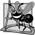## Software Engineering Observation 5 1

Experience has shown that the most difficult part of solving a problem on a computer is developing the algorithm for the solution. Once a correct algorithm has been specified, the process of producing a working C# application from the algorithm is normally straightforward.

Note the references in the algorithm of Fig. 5.5 to a total and a counter. A total is a variable used to accumulate the sum of several values. A counter is a variable used to countin this case, the grade counter indicates which of the 10 grades is about to be entered by the user. Variables used to store totals are normally initialized to zero before being used in an application.

Implementing Counter-Controlled Repetition in Class GradeBook

Class GradeBook (Fig. 5.6) contains a constructor (lines 1114) that assigns a value to the class's instance variable courseName (declared in line 8) by using property CourseName. Lines 1727 and 3035 declare property CourseName and method DisplayMessage, respectively. Lines 3864 declare method DetermineClassAverage, which implements the class-averaging algorithm described by the pseudocode in Fig. 5.5.

Lines 4043 declare local variables total, gradeCounter, grade and average to be of type int. Variable grade stores the user input.

Note that the declarations (in lines 4043) appear in the body of method DetermineClassAverage. Recall that variables declared in a method body are local variables and can be used only from the line of their declaration in the method to the closing right brace of the method declaration. A local variable's declaration must appear before the variable is used in that method. A local variable cannot be accessed outside the method in which it is declared.

In the versions of class GradeBook in this chapter, we simply read and process a set of grades. The averaging calculation is performed in method DetermineClassAverage using local variableswe do not preserve any information about student grades in instance variables of the class. In later versions of the class (in Chapter 8, Arrays), we maintain the grades in memory using an instance variable that refers to a data structure known as an array. This allows a GradeBook object to perform various calculations on the same set of grades without requiring the user to enter the grades multiple times.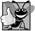## Good Programming Practice 5 6

Separate declarations from other statements in methods with a blank line for readability.

We say that a variable is definitely assigned when the variable is assigned in every possible flow of control. Notice that each local variable declared in lines 40-43 is definitely assigned before it is used in calculations. The assignments (in lines 4647) initialize total to 0 and gradeCounter to 1. Variables grade and average (for the user input and calculated average, respectively) need not be initialized heretheir values are assigned as they are input or calculated later in the method.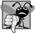## Common Programming Error 5 4

Using the value of a local variable before it is definitely assigned results in a compilation error. All local variables must be definitely assigned before their values are used in expressions.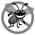## Error Prevention Tip 5 1

Initialize each counter and total, either in its declaration or in an assignment statement. Totals are normally initialized to 0. Counters are normally initialized to 0 or 1, depending on how they are used (we will show examples of each).

Line 50 indicates that the while statement should continue looping (also called iterating) as long as the value of gradeCounter is less than or equal to 10. While this condition remains true, the while statement repeatedly executes the statements between the braces that delimit its body (lines 5156).

Line 52 displays the prompt "Enter grade: " in the console window. Line 53 reads the grade entered by the user and assigns it to variable grade. Then line 54 adds the new grade entered by the user to the total and assigns the result to total, which replaces its previous value.

Line 55 adds 1 to gradeCounter to indicate that the application has processed a grade and is ready to input the next grade from the user. Incrementing gradeCounter eventually causes gradeCounter to exceed 10. At that point the while loop terminates because its condition (line 50) becomes false.

When the loop terminates, line 59 performs the averaging calculation and assigns its result to the variable average. Line 62 uses Console's WriteLine method to display the text "Total of all 10 grades is " followed by variable total's value. Line 63 then uses WriteLine to display the text "Class average is " followed by variable average's value. Method DetermineClassAverage returns control to the calling method (i.e., Main in GradeBookTest of Fig. 5.7) after reaching line 64.

Class GradeBookTest (Fig. 5.7) creates an object of class GradeBook (Fig. 5.6) and demonstrates its capabilities. Lines 910 of Fig. 5.7 create a new GradeBook object and assign it to variable myGradeBook. The string in line 10 is passed to the GradeBook constructor (lines 1114 of Fig. 5.6). Line 12 calls myGradeBook's DisplayMessage method to display a welcome message to the user. Line 13 then calls myGradeBook's DetermineClassAverage method to allow the user to enter 10 grades, for which the method then calculates and prints the averagethe method performs the algorithm shown in Fig. 5.5.

Notes on Integer Division and Truncation

The averaging calculation performed by method DetermineClassAverage in response to the method call at line 13 in Fig. 5.7 produces an integer result. The application's output indicates that the sum of the grade values in the sample execution is 848, which when divided by 10, should yield the floating-point number 84.8. However, the result of the calculation total / 10 (line 59 of Fig. 5.6) is the integer 84, because total and 10 are both integers. Dividing two integers results in integer divisionany fractional part of the calculation is lost (i.e., truncated, not rounded). We will see how to obtain a floating-point result from the averaging calculation in the next section.## Common Programming Error 5 5

Assuming that integer division rounds (rather than truncates) can lead to incorrect results. For example, 7 ÷ 4, which yields 1.75 in conventional arithmetic, truncates to 1 in integer arithmetic, rather than rounding to 2.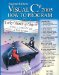Visual C# 2005 How to Program (2nd Edition)
ISBN: 0131525239
EAN: 2147483647
Year: 2004
Pages: 600

Similar book on Amazon